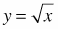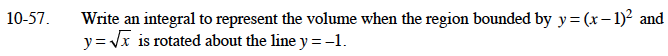### Home > CALC > Chapter Ch10 > Lesson 10.1.6 > Problem10-57

10-57.

Write an integral to represent the volume when the region bounded by y = (x − l)2 andis rotated about the line y = −1. Homework Help ✎Graph the functions to determine the points of intersection.

$R=1+\sqrt{x}$